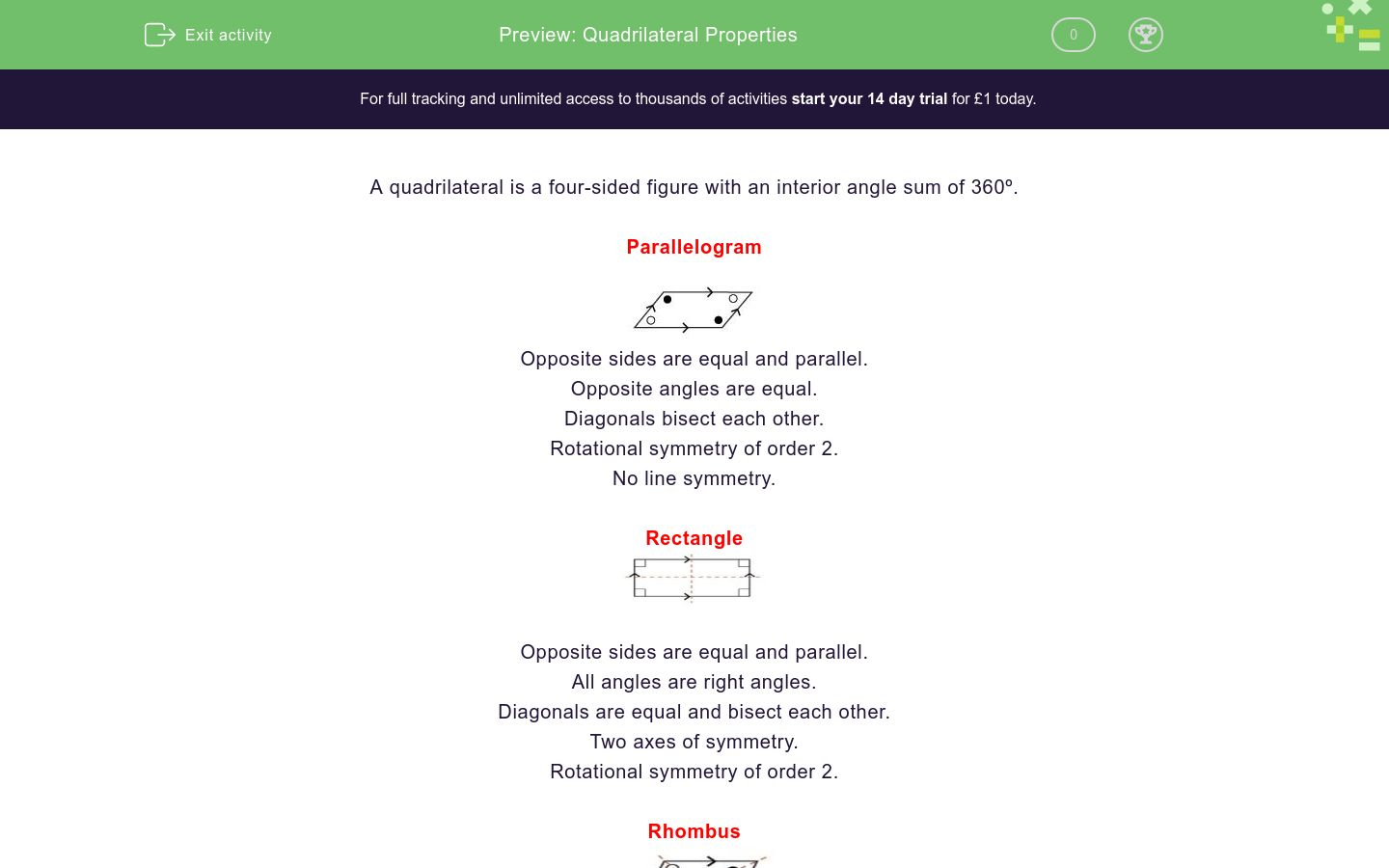### Affordable pricing from £10/month

In this worksheet, students remind themselves of the geometrical properties of quadrilaterals for their work with Pythagoras' Theorem.Key stage:  KS 4

Curriculum topic:  Geometry and Measures

Difficulty level:### QUESTION 1 of 10

A quadrilateral is a four-sided figure with an interior angle sum of 360º.

ParallelogramOpposite sides are equal and parallel.

Opposite angles are equal.

Diagonals bisect each other.

Rotational symmetry of order 2.

No line symmetry.

RectangleOpposite sides are equal and parallel.

All angles are right angles.

Diagonals are equal and bisect each other.

Two axes of symmetry.

Rotational symmetry of order 2.

RhombusAll sides are equal.

Opposite sides are parallel.

Opposite angles are equal.

Diagonals bisect each other at right angles.

Two axes of symmetry.

Rotational symmetry of order 2.

SquareAll sides are equal.

Opposite sides are parallel.

All angles are right angles.

Diagonals are equal and bisect each other at right angles.

Four axes of symmetry.

Rotational symmetry of order 4.

KiteTwo pairs of adjacent sides are equal.

Diagonals cut each other at right angles.

One diagonal is bisected by the other.

One axis of symmetry.

No rotational symmetry.

TrapeziumOne pair of opposite sides is parallel

Isosceles Trapezium

.One pair of opposite sides is parallel.

The non-parallel sides are equal.

Two pairs of adjacent equal angles.

One line of symmetry.

(In this worksheet, always give the most general answer.  This means that if a square and a rectangle fit the bill, you should write rectangle).

AB is parallel to CD and the diagonals are of equal length.

What type of quadrilateral is it?

Parallelogram

Rectangle

Rhombus

Square

Kite

Trapezium

Isosceles trapezium

AB is parallel to CD.  ∠A = ∠C.

What type of quadrilateral is it?

Parallelogram

Rectangle

Rhombus

Square

Kite

Trapezium

Isosceles trapezium

AB = BC and ∠A = ∠C = 90º.

What type of quadrilateral is it?

Parallelogram

Rectangle

Rhombus

Square

Kite

Trapezium

Isosceles trapezium

AC is a line of symmetry and ∠A = ∠C = 90º.

What type of quadrilateral is it?

Parallelogram

Rectangle

Rhombus

Square

Kite

Trapezium

Isosceles trapezium

AB is parallel to CD but AB ≠ CD. However BC = AD.

What type of quadrilateral is it?

Parallelogram

Rectangle

Rhombus

Square

Kite

Trapezium

Isosceles trapezium

ABCD is a parallelogram.

AC is perpendicular to BD but AC ≠ BD.

What type of quadrilateral is it?

Parallelogram

Rectangle

Rhombus

Square

Kite

Trapezium

Isosceles trapezium

ABCD is a parallelogram.

AC is not perpendicular to BD but AC = BD.

What type of quadrilateral is it?

Parallelogram

Rectangle

Rhombus

Square

Kite

Trapezium

Isosceles trapezium

ABCD is a parallelogram.

AC is perpendicular to BD and AC = BD.

What type of quadrilateral is it?

Parallelogram

Rectangle

Rhombus

Square

Kite

Trapezium

Isosceles trapezium

AC and BD cut each other at right angles at the point X, where AX = XC.

What type of quadrilateral is it?

Parallelogram

Rectangle

Rhombus

Square

Kite

Trapezium

Isosceles trapezium

Four congruent right-angled triangles are formed by the sides and the diagonals.

What type of quadrilateral is it?

Parallelogram

Rectangle

Rhombus

Square

Kite

Trapezium

Isosceles trapezium

• Question 1

AB is parallel to CD and the diagonals are of equal length.

What type of quadrilateral is it?

Isosceles trapezium
• Question 2

AB is parallel to CD.  ∠A = ∠C.

What type of quadrilateral is it?

Parallelogram
• Question 3

AB = BC and ∠A = ∠C = 90º.

What type of quadrilateral is it?

Kite
• Question 4

AC is a line of symmetry and ∠A = ∠C = 90º.

What type of quadrilateral is it?

Square
• Question 5

AB is parallel to CD but AB ≠ CD. However BC = AD.

What type of quadrilateral is it?

Isosceles trapezium
• Question 6

ABCD is a parallelogram.

AC is perpendicular to BD but AC ≠ BD.

What type of quadrilateral is it?

Rhombus
• Question 7

ABCD is a parallelogram.

AC is not perpendicular to BD but AC = BD.

What type of quadrilateral is it?

Rectangle
• Question 8

ABCD is a parallelogram.

AC is perpendicular to BD and AC = BD.

What type of quadrilateral is it?

Square
• Question 9

AC and BD cut each other at right angles at the point X, where AX = XC.

What type of quadrilateral is it?

Kite
• Question 10

Four congruent right-angled triangles are formed by the sides and the diagonals.

What type of quadrilateral is it?